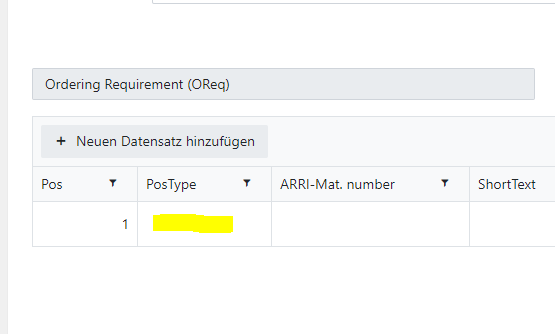# Continuous Automatic Position number in Data Table

Hi,

I want to add a continuous automatic position number after clicking on "Add New Record", so that I can get a sequence for all the positions.

Can anyone help me here?

Thank you.

Hello @kttg,

Welcome to Plumsail Community!

You can populate column value with the row number:

``````const dt = fd.control('Control0');

//get a column by its name
const countColumn = dt.columns.find(c => c.field === 'Column1');
countColumn.editable = () => false;

dt.widget.bind('beforeEdit', function(e) {
let model = e.model;
let count = clmnCount()
if (model.isNew()) {
model.set('Column1', count);
}
});

function clmnCount() {
return fd.control('Control0').widget.dataSource.data().length + 1
}
``````

Or you can change the column template to only display row number, the column value will be blank:

``````const dt = fd.control('Control0');
//get a column by its name
const countColumn = dt.columns.find(c => c.field === 'Column1');
countColumn.editable = () => false;

let columns = dt.widget.columns;
let record = 0;
columns = {
field: "Column1",
template: clmnTemplate,
attributes: {
style: "text-align: center"
},
width: 80
}

dt.widgetOptions = {
columns: columns
};
function clmnTemplate(data) {
return dt.widget.dataSource.indexOf(data) + 1
}``````

Hi,

I didn't get any of the both solutions to work. But I'm sure this is just because I'm still a rookie here.

I placed the solution within the fd.spRendered(function()) function.
First of all: Is this the right place for the code snippet?

In my data table I created a column of the Type Number named 'Pos':

This is the way I tried the first solution:

``````fd.spRendered(function(){
function clmnCount() {
return fd.control('DataTable1').widget.dataSource.data().length + 1
}
const dataTable = fd.control('DataTable1');

//get a column by its name
const countColumn = dt.columns.find(c => c.field === 'Pos');
countColumn.editable = () => false;

dt.widget.bind('beforeEdit', function(e) {
let model = e.model;
let count = clmnCount()
if (model.isNew()) {
model.set('Pos', count);
}
});

});
``````

I added the code snippet like the following for the second solution:

``````fd.spRendered(function() {
function clmnTemplate(data) {
return dt.widget.dataSource.indexOf(data) + 1
}

const dt = fd.control('DataTable1');
//get a column by its name
const countColumn = dt.columns.find(c => c.field === 'Pos');
countColumn.editable = () => false;

let columns = dt.widget.columns;
let record = 0;
columns = {
field: "Pos",
template: clmnTemplate,
attributes: {
style: "text-align: center"
},
width: 80
}

dt.widgetOptions = {
columns: columns
};
});
``````

What am I doing wrong here?

Yes, this is absolutely the right place for the code.

the code looks correct for me. Please check the browser console (F12) for errors and share the screenshot.

Please export the form and share it either here or send to [email protected]:I'll send it to you via the support email.
Thank you

Dear Plumsail team,

Thank you so much for your help. This is the solution that works. I added the -1 so that the Pos number starts correct:

``````  function clmnCount() {
return fd.control('DataTable1').widget.dataSource.data().length + 1
}

const dt = fd.control('DataTable1');

//get a column by its name
const countColumn = dt.columns.find(c => c.field === 'Pos');
countColumn.editable = () => false;

dt.widget.bind('beforeEdit', function(e) {
let model = e.model;
let count = clmnCount()
if (model.isNew()) {
model.set('Pos', count - 1
);
}
});

});
``````
1 Like

Dear mnikitina,

I found another issue. Maybe you can also help me with it.

After clicking on add new dataset to table, the Pos number is not directly filled:

The value for the Pos column gets a value as soon as I click into another column of that row:Do you know a solution with which the Pos value will directly be filled after clicking on add new dataset to table?

Thank you so much in advance!

Try this code:

``````function clmnCount() {
return fd.control('DataTable1').widget.dataSource.data().length + 1
}

//select the DataTable control to automatically populate new rows
var dt = fd.control('DataTable1');
//get a column by its name
const countColumn = dt.columns.find(c => c.field === 'Pos');
countColumn.editable = () => false;

dt.\$on('change', function(value) {
if (value) {
// go through each row one by one
for (var i = 0; i < value.length; i++) {
value[i].set('Pos', i + 1);

}
}
});``````

Hi mnikitina,

this solution works great!

Thank you so much!

1 Like